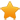Switch Editions?
CancelChannel: CodeSection,代码区,网络安全 - CodeSec
Viewing all articles

# 关于C语言不定参数的研究

0
0

Showchar 函数的内容：

（1）main 函数将 char 型数据和 int 型数据入栈是占 2 个字节，那么如果是 float 型或者 longint 型、 double 型、 longdouble 型等超过 2 字节的变量类型怎么办？

（2）Showchar 函数将栈中取出的参数赋给 al ，为什么 2 是 int 型也只赋给一个字节的 al ？如果是更大的参数怎么办？

（3）我们注意到这一个指令

Showchar 函数为：

Showchar 函数为：

void printf(char *,...);

int pow(int, int);

main()

{

/*printf("I think this is interesting :%c and %c and %c",0x61,0x62,0x63);*/

printf("No.%d,%d,%d,this is me %c ing yuan",45,123,8958,'Q');

}

void printf(char *des, ...)

{

/*first sure the length of string des*/

int len=0;

int i=0;

int showp=0; /*define the point of showing chars*/

int parap=0; /*define of parameter position in stack*/

int intValueLength=1;

int signalNum=0;

/*calculate length of stirng des */

while(des[i]!='/0')

{

len++;

i++;

}

i=0;

while(des[i]!='/0')

{

if(des[i]=='%')

{

/*check type of value user want to show.*/

if(des[i+1]=='d')

{

/*here show integer value*/

int showIntValue=*(int *)(_BP+6+parap+parap); /*here, we show understand that define one char point value, we just push the point value into stack, but not the string value*/

int reValue=showIntValue;

/* *(int far *)(0xb8000000+160*10+80+showp+showp)=showIntValue;

*(int far *)(0xb8000000+160*10+81+showp+showp)=2;*/

i+=2;

parap++;

intValueLength=1;

/*here we calculate the length of integer value we want to show ,and then we can sure the next value show position*/

while(reValue/10!=0)

{

intValueLength++;

reValue/=10;

}

/*first calculate the length of unmber and show every sigal positon number of Integer *

Viewing all articles

### 【串烧】十一月最新Club.House.Megamix.200个[28G/DJ.Tits]

More Pages to Explore .....